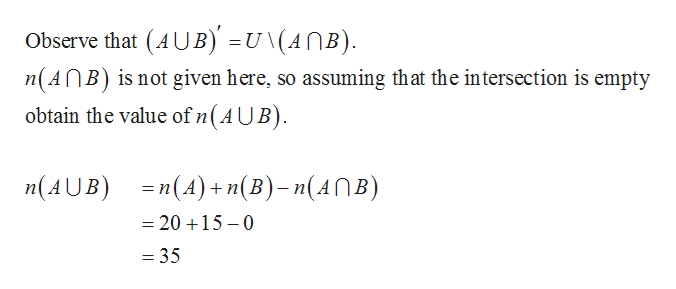# Assume that n(U)=70n(U)=70, n(A)=20n(A)=20, and n(B)=15n(B)=15.Then n((A∪B)′)=

Question

Assume that n(U)=70n(U)=70, n(A)=20n(A)=20, and n(B)=15n(B)=15.

Then n((A∪B)′)=

check_circleExpert Solution
Step 1

The given details are

n(U) = 70

&n...help_outlineImage TranscriptioncloseObserve that (AUB) =u \(AnB) n(AnB) is not given here, so assuming that the intersection is empty obtain the value of n(AUB) nAUB)(A)+n(Bn(ANB) =20 15-0 = 35 fullscreen

### Want to see the full answer?

See Solution

#### Want to see this answer and more?

Solutions are written by subject experts who are available 24/7. Questions are typically answered within 1 hour*

See Solution
*Response times may vary by subject and question
Tagged in

### Math